Take the Last leap| Aakash BYJU'S JEE (Adv) '23 Mock Test on 28th May Take the Last leap| Aakash BYJU'S JEE (Adv) '23 Mock Test on 28th May

# JEE Main 2022 June 27 – Shift 2 Chemistry Question Paper with Solutions

The JEE Main 2022 June 27 Shift 2 Chemistry question paper with solutions is available on this page. Our subject experts have provided solutions for all the JEE main 2022 question papers to help students enhance their preparation for the upcoming exams. The solutions for the JEE main 2022 chemistry question paper can be accessed here. You can also get a printable pdf for the question papers of JEE main 2022. Go through the detailed solutions for the June 27 second shift JEE main 2022 chemistry question paper below.

## JEE Main 2022 June 27th Chemistry Shift 2 Question Paper and Solutions

#### SECTION – A

Multiple Choice Questions: This section contains 20 multiple choice questions. Each question has 4 choices (1), (2), (3) and (4), out of which ONLY ONE is correct.

1. Which amongst the given plots is the correct plot for pressure (p) vs density (d) for an ideal gas?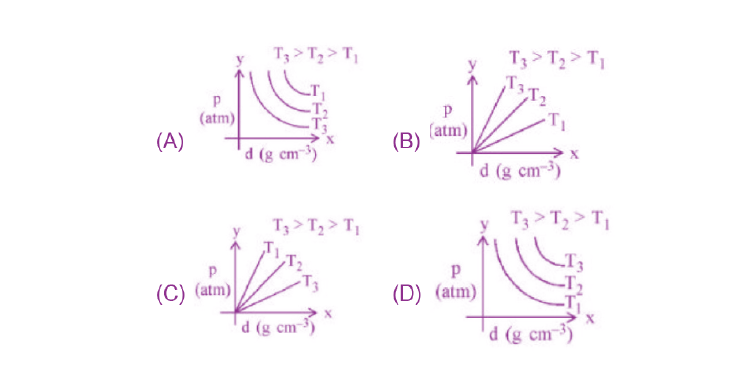Sol.

$$\begin{array}{l}\because d = \frac{pM}{RT}\end{array}$$

Hence, dRT = pM

p ∝ T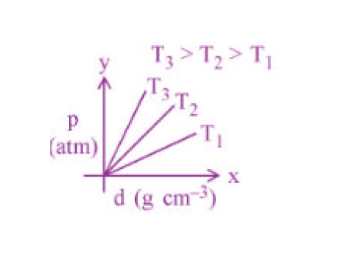2. Identify the incorrect statement for PCI5 from the following.

(A) In this molecule, orbitals of phosphorous are assumed to undergo sp3d hybridization.

(B) The geometry of PCl5 is trigonal bipyramidal.

(C) PCl5 has two axial bonds stronger than three equatorial bonds.

(D) The three equatorial bonds of PCl5 lie in a plane

Sol. PCl5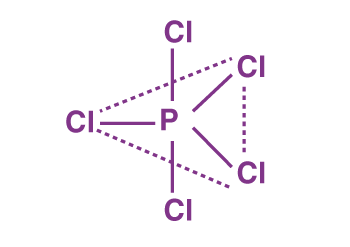● All three equatorial bonds in a plane

sp3d hybridization

● Trigonal bipyramidal

● Axial bonds are weaker than equatorial bonds.

3. Statement-I : Leaching of gold with cyanide ion in absence of air/O2 leads to cyano complex of Au(III).

Statement-II : Zinc is oxidized during the displacement reaction carried out for gold extraction.

In the light of the above statements, choose the correct answer from the options given below.

(A) Both statement-I and statement-II are correct

(B) Both statement-I and statement-II are incorrect

(C) Statement-I is correct but statement-II is incorrect

(D) Statement-I is incorrect but statement-II is correct

Sol. Leaching of gold with cyanide ion is done in presence of air/O2 leading to cyano complex [Au(CN)2] where Au is in +1 oxidation state.

$$\begin{array}{l}4Au(s)+8CN^-(aq)+2H_2O(aq)+O_2(g)\longrightarrow 4\left[Au(CN)_2\right]_{aq}^-+4OH^-\end{array}$$
$$\begin{array}{l}2\left[Au(CN)_2\right]_{aq}^-+Zn(s)\longrightarrow \left[Zn(CN)_4\right]_{aq}^{2-}+2Au(s) \end{array}$$

Zinc is oxidised from (0) to +2 oxidation state during displacement reaction carried out for gold extraction.

4. The correct order of increasing intermolecular hydrogen bond strength is

(A) HCN < H2O < NH3

(B) HCN < CH4 < NH3

(C) CH4 < HCN < NH3

(D) CH4 < NH3 < HCN

Sol. Due to high difference in electronegativity of H and N the H-bond strength of NH3 is highest. There is no H-bond in CH4.

CH4 < HCN < NH3

Hence, correct option is (C)

5. The correct order of increasing ionic radii is

(A) Mg2+ < Na+ < F < O2– < N3–

(B) N3– < O2– < F < Na+ < Mg2+

(C) F < Na+ < O2– < Mg2+ < N3–

(D) Na+ < F< Mg2+ < O2– < N3–

Sol. For isoelectronic species

$$\begin{array}{l}\text{Ionic radii}\propto \frac{\text{(-)ve charge}}{\text{(+)ve charge}}\end{array}$$

Hence, correct order of ionic radii is

Mg2+ < Na+ < F < O2– < N3–

6. The gas produced by treating an aqueous solution of ammonium chloride with sodium nitrite is

(A) NH3

(B) N2

(C) N2O

(D) Cl2

Sol. N2 gas is produced by treating an aqueous solution of ammonium chloride with sodium nitrite.

$$\begin{array}{l}\underset{\text{Ammonium} \\ \text{ chloride}}{NH_4Cl}(aq) + \underset{\text{Sodium}\\ \text{ nitrite}}{NaNo_2}(aq)\longrightarrow \underset{\text{Nitrogen} \\ \text{ gas}}{N_2}(g)+\underset{\text{Water}\\}{2H_2O}(l)+\underset{\text{Sodium \\ ~\text{chloride}}}{NaCl}(aq) \end{array}$$

7. Given below are two statements: one is labelled as Assertion A and the other is labelled as Reason R.

Assertion A: Fluorine forms one oxoacid.

Reason R: Fluorine has smallest size amongst all halogens and is highly electronegative.

In the light of the above statements, choose the most appropriate answer from the option given below.

(1) Both A and R are correct and R is the correct explanation of A.

(2) Both A and R are correct but R is NOT the correct explanation of A.

(3) A is correct but R is not correct.

(4) A is not correct but R is correct.

Sol. Due to smaller size, fluorine forms only one oxoacid.

Both the Assertion and Reason are correct and Reason is the correct explanation.

8. In 3d series, the metal having the highest M2+/M standard electrode potential is

(A) Cr

(B) Fe

(C) Cu

(D) Zn

Sol. Metal E° M2+/M

Cr –0.90 V

Fe –0.44 V

Cu + 0.34 V

Zn (–0.76 V)

The metal having highest E°(M2+/M) standard reduction potential is Cu.

9. The ′f′ orbitals are half and completely filled, respectively in lanthanide ions

[Given: Atomic no. Eu, 63; Sm, 62; Tm, 69; Tb, 65; Yb, 70; Dy, 66]

(A) Eu2+ and Tm2+

(B) Sm2+ and Tm3+

(C) Tb4+ and Yb2+

(D) Dy3+ and Yb3+

Sol.

 +2 +3 +4 Eu 4f7 4f6 Tm 4f13 4f12 Sm 4f6 4f5 Tb 4f9 4f8 4f7 Yb 4f14 4f13 Dy 4f10 4f9

Hence, the pair Tb+4 Yb+2 have half filled and completely filled f subshells respectively.

10. Arrange the following coordination compounds in the increasing order of magnetic moments. (Atomic numbers: Mn = 25; Fe = 26)

(1) [FeF6]3–

(2) [Fe(CN)6]3–

(3) [MnCl6]3– (high spin)

(4) [Mn(CN)6]3–

Choose the correct answer from the options given below:

(A) 1 < 2 < 4 < 3

(B) 2 < 4 < 3 < 1

(C) 1 < 3 < 4 < 2

(D) 2 < 4 < 1 < 3

Sol.

 Coordination Compound Number of unpaired e– (n) Magnetic moment (μ) (B.M) A [FeF6]3– – d5 5 5.91 B [Fe(CN)6]3– – d5 1 1.73 C [MnCl6]3– – d4 4 4.89 D [Mn(CN)6]3– – d4 2 2.82

Hence, the correct order of magnetic moment is

2 < 4 < 3 < 1

11. On the surface of polar stratospheric clouds, hydrolysis of chlorine nitrate gives A and B while its reaction with HCl produces B and C. A, B and C are, respectively

(A) HOCl, HNO3, Cl2

(B) Cl2, HNO3, HOCl

(C) HClO2, HNO2, HOCl

(D) HOCl, HNO2, Cl2O

Sol. On the surface of polar stratospheric clouds, hydrolysis of chlorine nitrate as

$$\begin{array}{l}ClONO_2+H_2O\longrightarrow \underset{A}{HOCl}+\underset{B}{HNO_3}\end{array}$$
$$\begin{array}{l}ClONO_2+HCl\longrightarrow \underset{C}{Cl_2}+\underset{B}{HNO_3} \end{array}$$

Hence A, B and C are HOCl, HNO3 and Cl2, respectively.

12. Which of the following is most stable?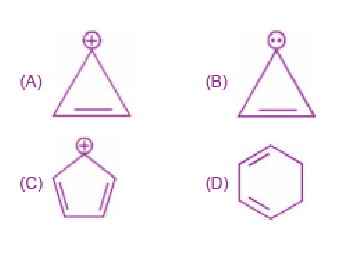Sol.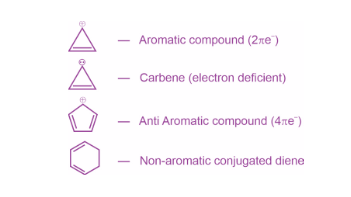1,3-cyclohexadiene is most stable because it is a neutral molecule. All others are intermediates and hence less stable.

13. What will be the major product of the following sequence of reactions?

$$\begin{array}{l}n-Bu-\equiv \xrightarrow[\text{(ii) Lindlar catalyst, }H_2]{(i) n- BuLi, \\ n-C_5H_{11}Cl}\end{array}$$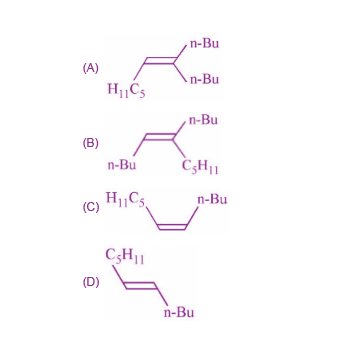Sol.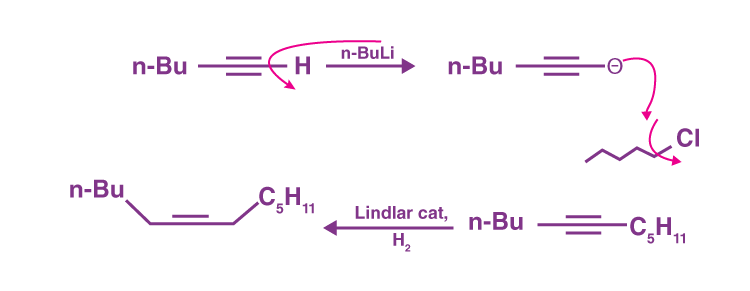Hence, the correct option is (C).

14. Product ‘A’ of the following sequence of reactions is

$$\begin{array}{l}\text{Ethylbenzene} \xrightarrow[\text{(b)}Cl_2, \Delta; \\ \text{(c) alc.}KOH]{\text{(a)}Br_2, Fe} \underset{\text{major Product}}{‘A’}\end{array}$$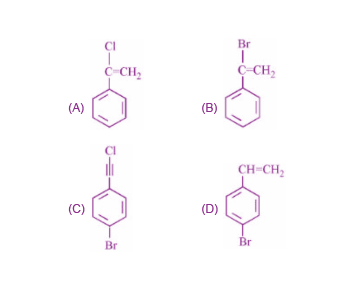Sol.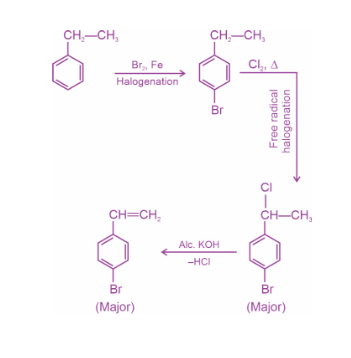15. Match List I with List II.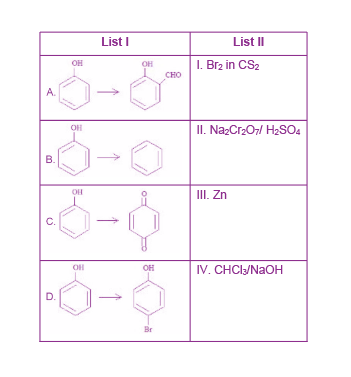Choose the correct answer from the options given below:

(A) A-IV, B-III, C-II, D-I

(B) A-IV, B-III, C-I, D-II

(C) A-II, B-III, C-I, D-IV

(D) A-IV, B-II, C-III, D-I

Sol.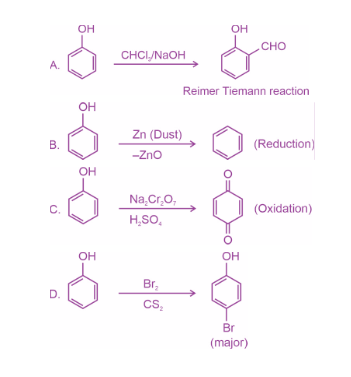∴Correct match is

(A) – IV, (B) – III, (C) – II, (D) – I

16. Decarboxylation of all six possible forms of diaminobenzoic acid C6H3(NH2)2COOH yields three products A, B and C. Three acids give a product ‘A’, two acids give a product ‘B’ and one acid gives a product ‘C’. The melting point of product ‘C’ is

(A) 63ºC

(B) 90ºC

(C) 104ºC

(D) 142ºC

Sol. The six possible forms of diaminobenzoic acid are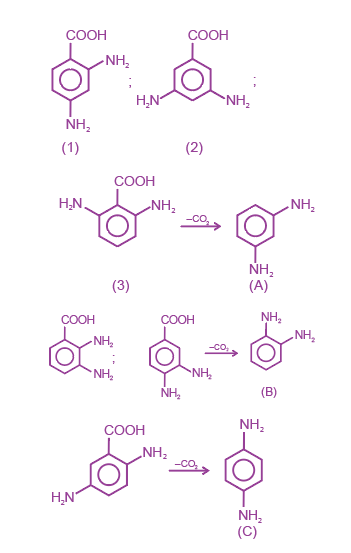Melting point of product (C) = 142ºC

17. Which is true about Buna-N?

(A) It is a linear polymer of 1, 3-butadiene

(B) It is obtained by copolymerization of 1, 3-butadiene and styrene

(C) It is obtained by copolymerization of 1, 3-butadiene and acrylonitrile

(D) The suffix N in Buna-N stands for its natural occurrence.

Sol. Buna-N is formed by copolymerisation of 1-3-butadiene and acrylonitrile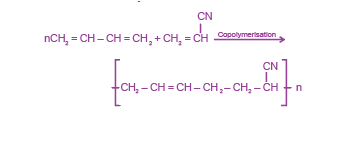18. Given below are two statements

Statement I: Maltose has two α-D-glucose units linked at C1 and C4 and is a reducing sugar.

Statement II: Maltose has two monosaccharides:

α-D-glucose and β-D-glucose linked at C1 and C6 and it is a non-reducing sugar.

In the light of the above statements, choose the correct answer from the options given below.

(A) Both Statement I and Statement II are true

(B) Both Statement I and Statement II are false

(C) Statement I is true but Statement II is false

(D) Statement I is false but Statement II is true

Sol. Maltose is composed of two α-D-glucose units in which C1 of one glucose unit and C4 of second glucose unit are linked.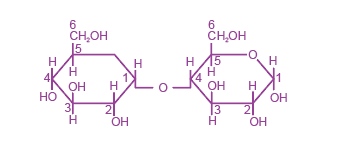19. Match List I with List II.

 List I List II A. Antipyretic I. Reduces pain B. Analgesic II. Reduces stress C. Tranquilizer III. Reduces fever D. Antacid IV. Reduces acidity (stomach)

Choose the correct answer from the options given below:

(A) A-III, B-I, C-II, D-IV

(B) A-III, B-I, C-IV, D-II

(C) A-I, B-IV, C-II, D-III

(D) A-I, B-III, C-II, D-IV

Sol. Antipyretic – Reduces fever

Analgesic – Reduces pain

Tranquilizer – Reduces stress

Antacid – Reduces Acidity (stomach)

20. Match List I with List II.

 List I (Anion) List II (gas evolved on reaction with dil H2SO4) A. $$\begin{array}{l}CO_3^{2-}\end{array}$$ I. Colourless gas which turns lead acetate paper black. B. S2– II. Colourless gas which turns acidified potassium dichromate solution green C. $$\begin{array}{l}SO_3^{2-}\end{array}$$ III. Brown fumes which turns acidified KI solution containing starch blue. D. $$\begin{array}{l}NO_2^{-}\end{array}$$ IV. Colourless gas evolved with brisk effervescence, which turns lime water milky.

Choose the correct answer from the options given below:

(A) A-III, B-I, C-II, D-IV (B) A-II, B-I, C-IV, D-III

(C) A-IV, B-I, C-III, D-II (D) A-IV, B-I, C-II, D-III

Sol.

$$\begin{array}{l}CO_3^{2-}:\end{array}$$
On action of dil sulphuric acid, CO2 gas is released which turns lime water milky.

S2– : On action of dil sulphuric acid, H2S gas is released which turns lead acetate paper black.

$$\begin{array}{l}SO_3^{2-}:\end{array}$$
On action of dil H2SO4, SO2 gas is evolved which turns acidified potassium dichromate solution green.

$$\begin{array}{l}NO_2^{-}:\end{array}$$
On action of dil H2SO4, NO2 gas is evolved which turns Kl solution containg starch blue.

#### SECTION – B

Numerical Value Type Questions: This section contains 10 questions. In Section B, attempt any five questions out of 10. The answer to each question is a NUMERICAL VALUE. For each question, enter the correct numerical value (in decimal notation, truncated/rounded-off to the second decimal place; e.g. 06.25, 07.00, –00.33, –00.30, 30.27, –27.30) using the mouse and the on-screen virtual numeric keypad in the place designated to enter the answer.

1. 116 g of a substance upon dissociation reaction, yields 7.5 g of hydrogen, 60 g of oxygen and 48.5 g of carbon. Given that the atomic masses of H, O and C are 1, 16 and 12, respectively. The data agrees with how many formulae of the following? _______.

A. CH3COOH

B. HCHO

C. CH3OOCH3

D. CH3CHO

Sol.

 Element Mass% Moles% Relative moles H 6.46 6.46 2 O 51.72 3.23 1 C 41.81 3.48 1

∴ Empirical formula = COH2

The empirical formula goes with acetic acid CH3COOH and formaldehyde HCHO.

Thus data agrees with 2 formulae.

2. Consider the following set of quantum numbers.

n l ml

A. 3 3 –3

B. 3 2 –2

C. 2 1 +1

D. 2 2 +2

The number of correct sets of quantum numbers is _____.

Sol. The correct sets of Quantum numbers are, (02)

n = 3 l = 2 ml = –2

and n = 2 l = 1 ml = +1

I can have values from 0 to (n – 1) and m can have values from –l….. 0 ……+l (2l + 1)

3. BeO reacts with HF in presence of ammonia to give [A] which on thermal decomposition produces [B] and ammonium fluoride. Oxidation state of Be in [A] is _______

Sol.

$$\begin{array}{l}BeO+2NH_3+4HF \longrightarrow \underset{(A)}{(NH_4)_2[BeF_4]} \end{array}$$
$$\begin{array}{l}(NH_4)_2(BeF_4)\xrightarrow[]{\text{Heating}} \underset{(B)}{BeF_2}+2NH_4F\end{array}$$

Oxidation State of Be in (A) is (+2)

4. When 5 moles of He gas expand isothermally and reversibly at 300 K from 10 litre to 20 litre, the magnitude of the maximum work obtained is _____ J. [nearest integer] (Given : R = 8.3 J K–1 mol–1 and log 2 = 0.3010)

Sol. Wrev = –2.303 nRT log10 (V2/V1)

= –2.303 × 5 × 8.3 × 300 × log10(20/10)

≈ –8630 J

5. A solution containing 2.5 × 10–3 kg of a solute dissolved in 75 × 10–3 kg of water boils at 373.535 K. The molar mass of the solute is _____ g mol–1. [nearest integer] (Given : Kb(H2O) = 0.52 K kg mol–1 and boiling point of water = 373.15 K)

Sol. Wsolute = 2.5 × 10–3 kg

Wsolvent = 75 × 10–3 kg

ΔTb = 373.535 – 373.15

= 0.385 K

Kb(H2O) = 0.52 K kg mol–1

$$\begin{array}{l}\triangle T_b=\frac{K_b \times 10^3 \times \text{W}_{\text{solute}}}{\text{M}_{\text{solute}}\times \text{W}_{\text{solvent}}} \end{array}$$
$$\begin{array}{l}\text{M}_{\text{solute}}=\frac{0.52\times 10^3 \times2.5 \times 10^{-3}}{75 \times 10^{-3}\times 0.385} \end{array}$$

= 45.02

≈ 45

6. pH value of 0.001 M NaOH solution is________.

Sol. [OH] = 0.001 = 10–3 M

[H+] [OH] = 10–14

[H+] = 10–11

pH = –log[H+]

= –log (10–11)

pH = 11

7. For the reaction taking place in the cell:

Pt(s) | H2(g) | H+ (q) || Ag+(aq) | Ag(s)

EoCell = + 0.5332 V

The value of ΔfG is _____kJ mol–1 [in nearest integer]

Sol. Pt(s)|H2(g) H+ (aq)||Ag+(aq)|Ag(s)

$$\begin{array}{l}\frac{1}{2} H_2 + Ag^{+}\rightarrow Ag + H^{+}\end{array}$$

n = 1

EoCell = 0.5332

ΔG° = –nFE°

= – 1 × 96500 × 0.5332

= – 51.453 kJ/mole

≃ 51 kJ/mole

8. It has been found that for a chemical reaction with rise in temperature by 9 K the rate constant gets doubled. Assuming a reaction to be occurring at 300 K, the value of activation energy is found to be _________kJ mol–1. [nearest integer]

(Given ln10 = 2.3, R = 8.3 J K–1 mol–1, log 2 = 0.30)

Sol. T1 = 300 K

(Rate constant)

K2 = 2K1, on increase temperature by 9K

T2 = 309 K

Ea = ?

$$\begin{array}{l}log \frac{K_2}{K_1} = \frac{Ea}{2.3 R} \left [ \frac{T_2-T_1}{T_2.T_1} \right ]\end{array}$$
$$\begin{array}{l}\textup{log 2}=\frac{Fa}{2.3 \times 8.3} \left [ \frac{9}{309 \times 300} \right ]\end{array}$$
l

$$\begin{array}{l}\text E_a = \frac{0.3 \times 309 \times 300 \times 2.3 \times 8.3}{9}\end{array}$$

= 58988.1 J/mole

≃ 59 kJ/mole

9.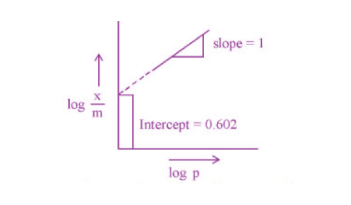If the initial pressure of a gas 0.03 atm, the mass of the gas absorbed per gram of the adsorbent is ____× 10–2g.

Sol. Given that log K = intercept = 0.602 = log 4

∴ K = 4

Slope

$$\begin{array}{l}=\frac{1}{n} = 1\end{array}$$

and initial pressure = 0.03 atm

$$\begin{array}{l}\frac{x}{m}=K(p)^{\frac{1}{n}}\end{array}$$

= 4 × 0.03 = 0.12 = 12 × 10–2

∴ mass of gas absorbed per gm of adsorbent

= 12 × 10–2 g

10. 0.25 g of an organic compound containing chlorine gave 0.40 g of silver chloride in Carius estimation. The percentage of chlorine present in the compound is ______. [in nearest integer]

(Given : Molar mass of Ag is 108 g mol–1 and that of Cl is 35.5 g mol–1)

Sol. Mass of organic compound = 0.25 g

Mass of AgCl = 0.40 g

$$\begin{array}{l}\%Cl=\frac{35.5 \times \text{mass of }AgCl}{143.5 \times \text{(mass of organic compound)}}\times 100 \end{array}$$
$$\begin{array}{l}=\frac{35.5 \times 0.40 \times 100}{143.5 \times 0.25}\end{array}$$

= 39.581

≃ 40

% Cl = 40 %

### Download PDF of JEE Main 2022 June 27 Shift 2 Chemistry Paper & Solutions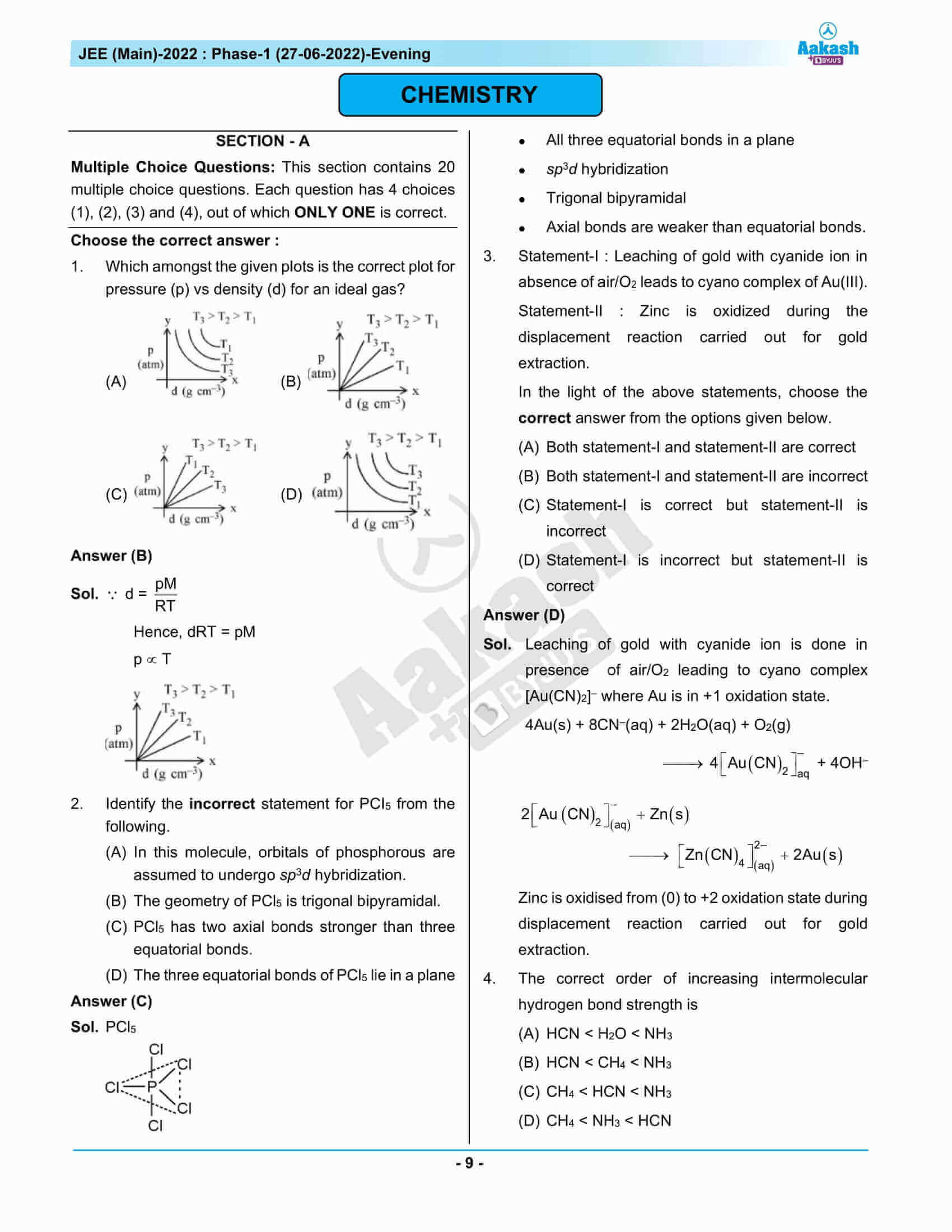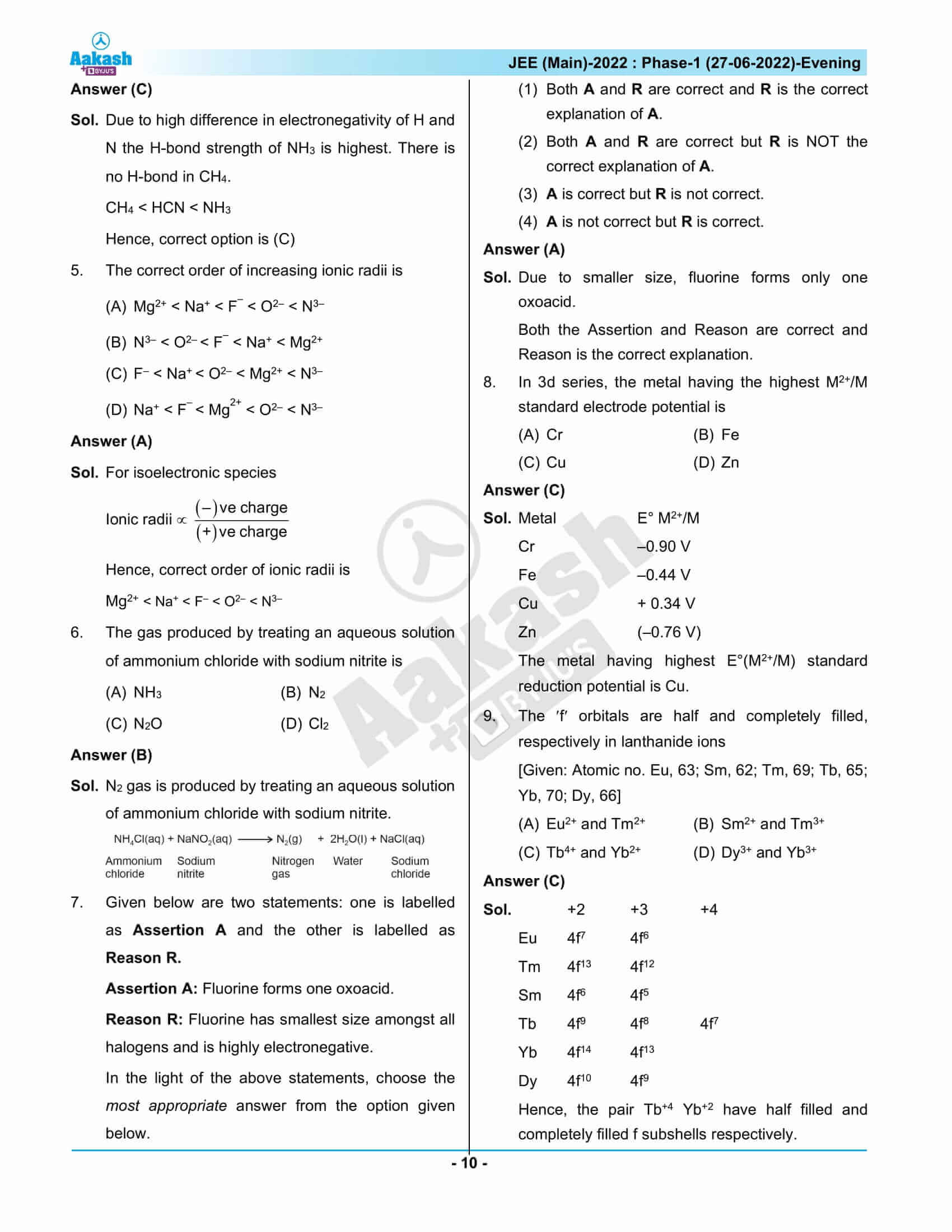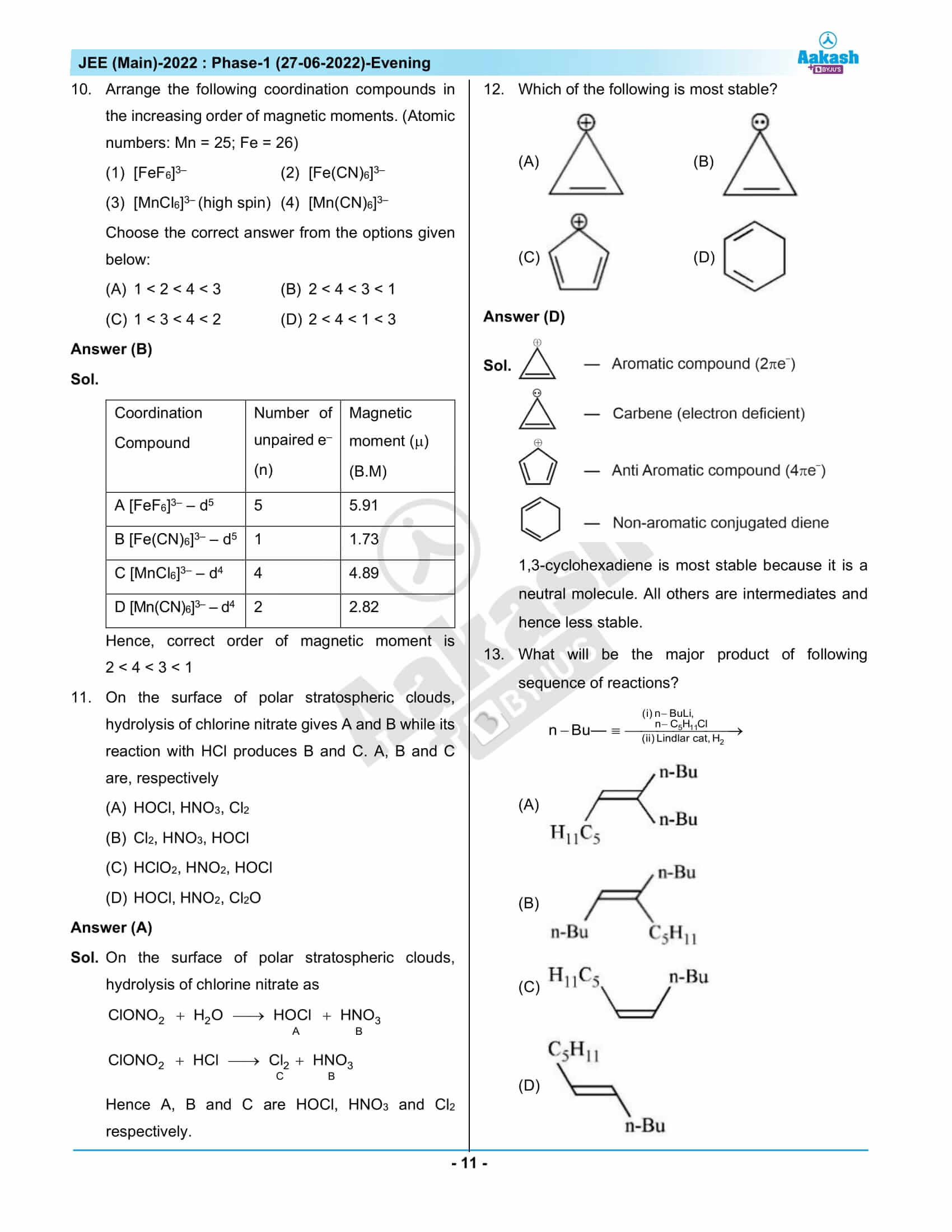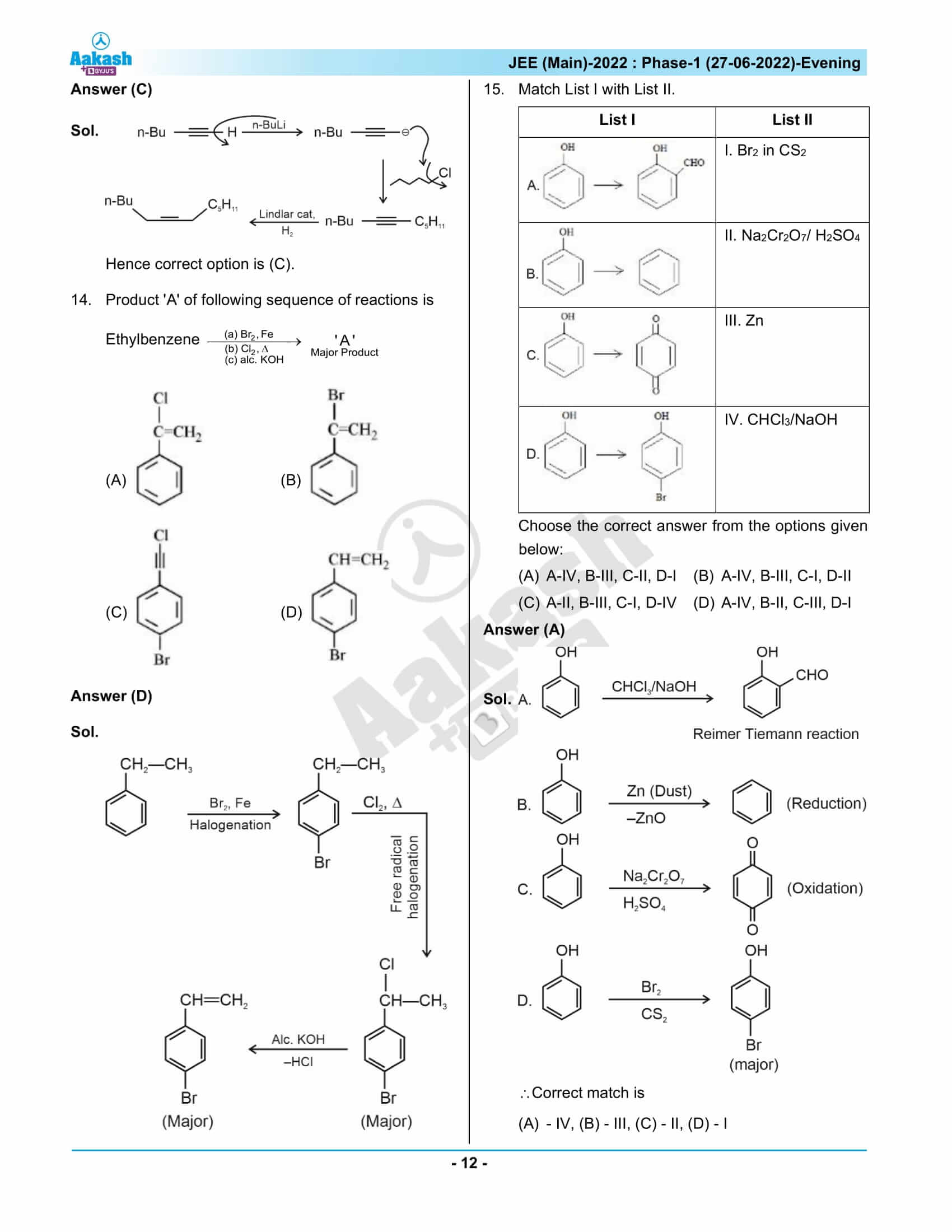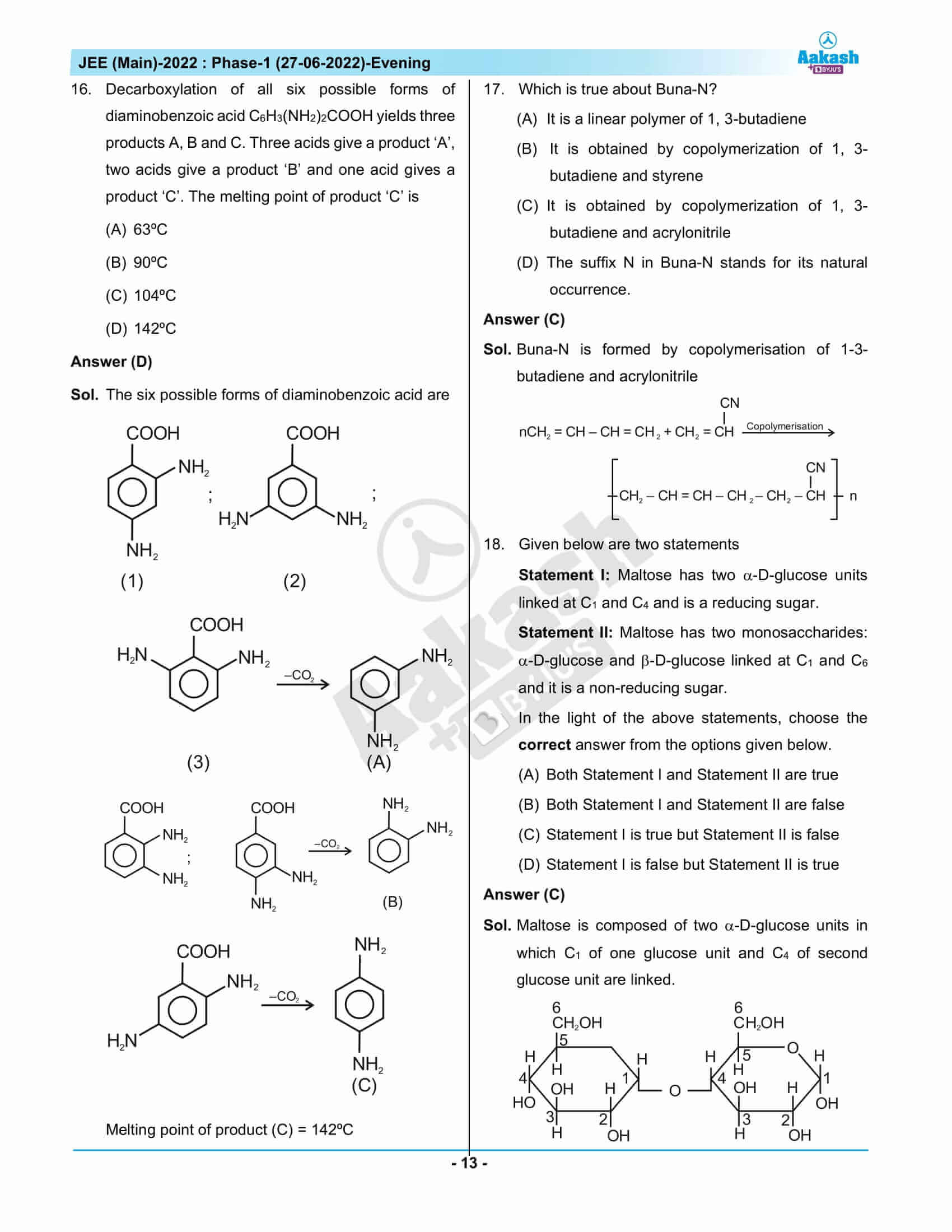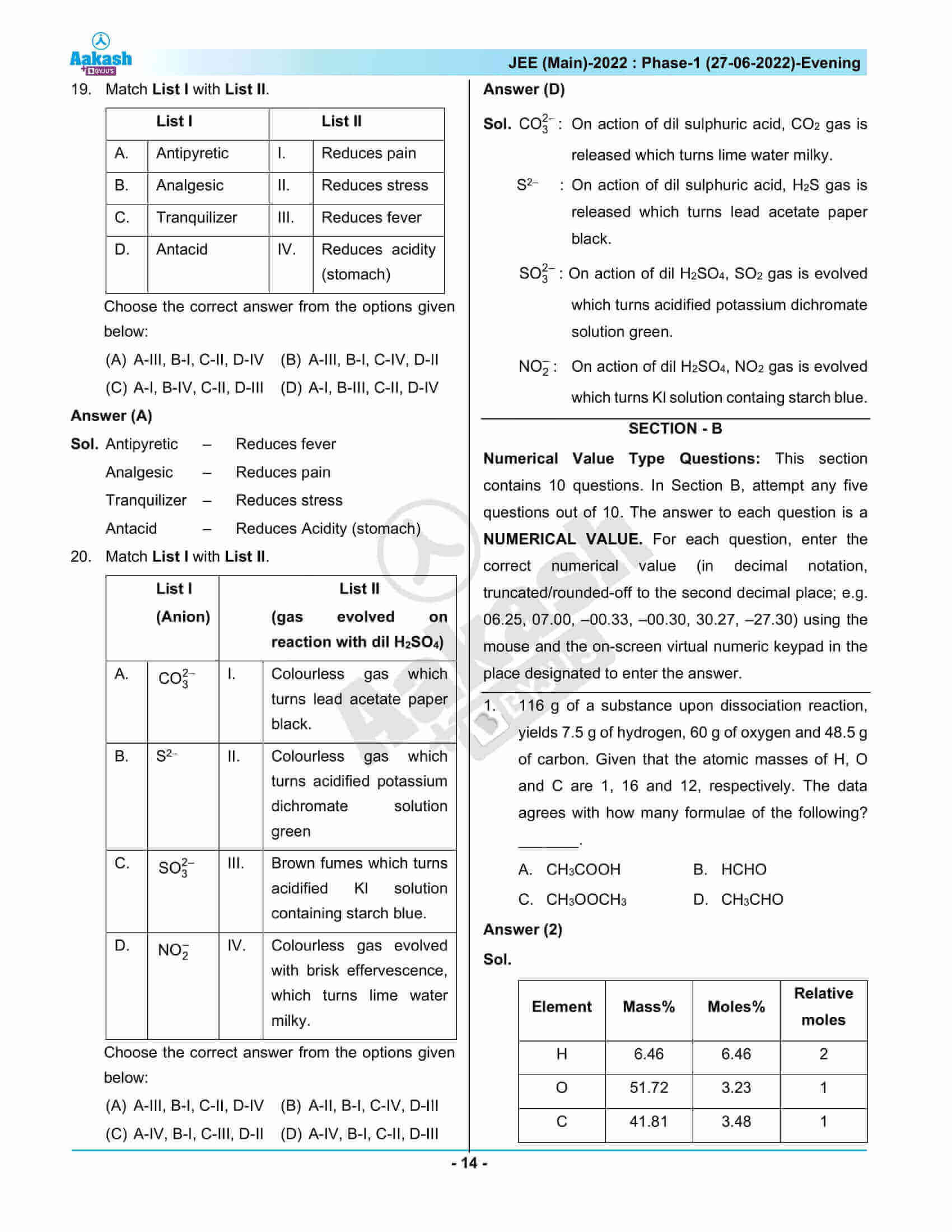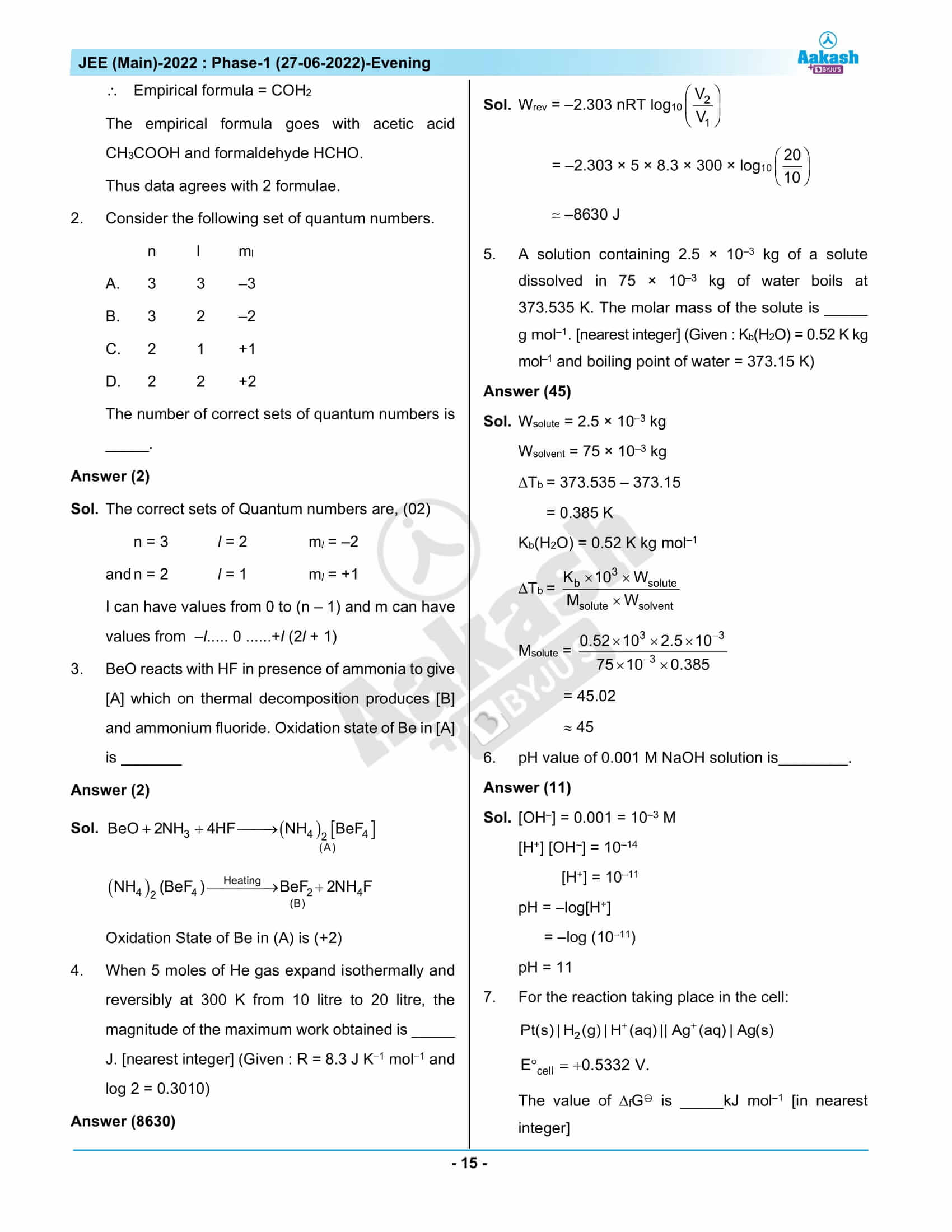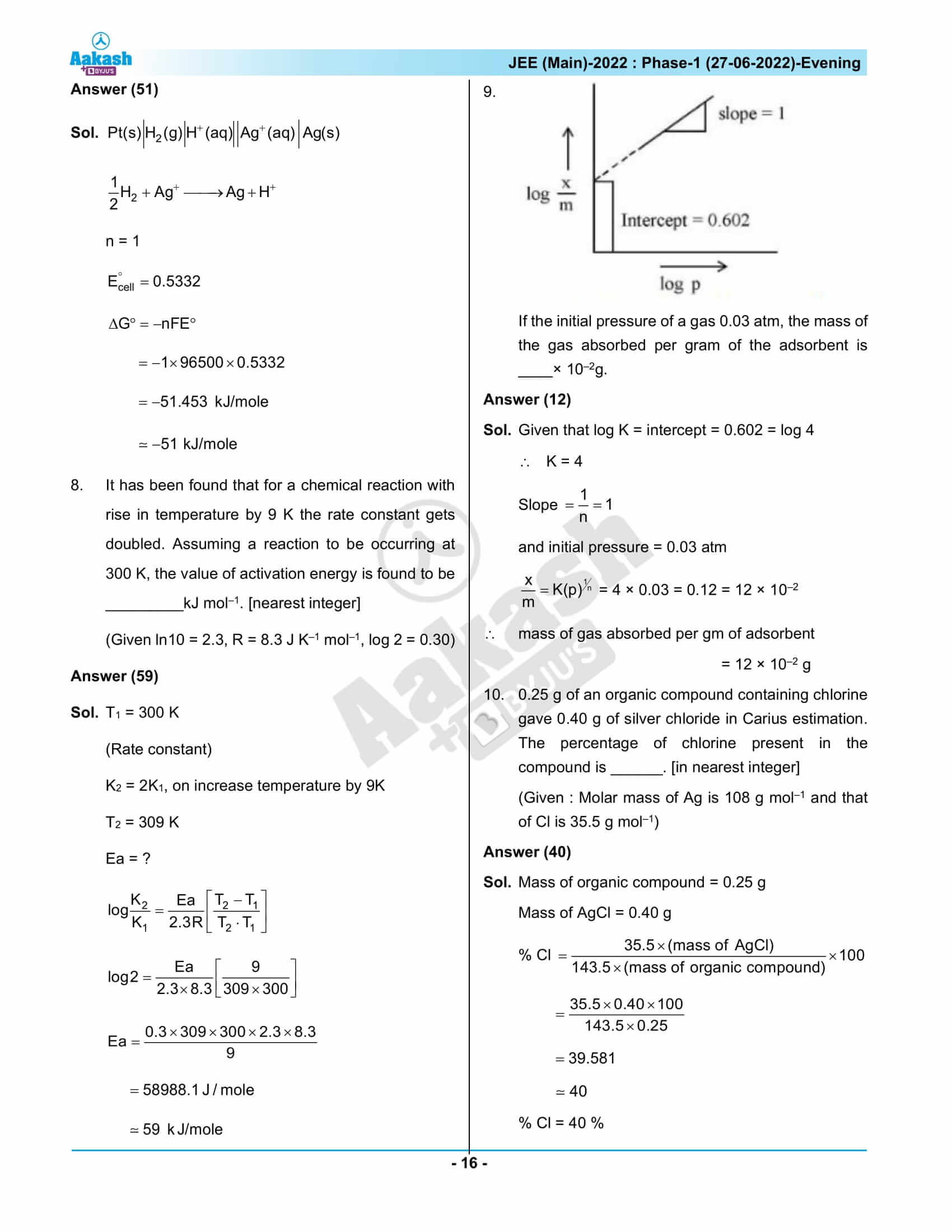## JEE Main 2022 June 27th Shift 2 Question Paper & Solutions

### JEE Main 2022 June 27 Shift 2 Question Paper – Physics Solutions### JEE Main 2022 June 27 Shift 2 Question Paper – Chemistry Solutions### JEE Main 2022 June 27 Shift 2 Question Paper – Maths Solutions## Frequently Asked Questions – FAQs

Q1

### List a few Chemistry topics from which easy questions were asked in JEE Main 2022 June 27 shift 2 question paper.

Some of the Chemistry topics from which easy questions were asked in JEE Main 2022 June 27 shift 2 were:
Atmospheric Pollution
Chemical Reaction of Phenols
Therapeutic Action of Different Classes of Drugs
Rubber
Calculation of PH
Aromaticity
Quantum Mechanical Model of The Atom
Dinitrogen
The Gas Laws
Bohr’s Model For Hydrogen Atom

Q2

### What were the Class 12 Chemistry chapters from which questions were included in JEE Main 2022 June 27, shift 2 question paper?

The chapters from which questions were asked in the Class 12 Chemistry syllabus were:
Alcohols, Phenols and Ethers
Principles of Qualitative Analysis
Coordination Compounds
General Principles and Processes of Isolation of Elements
Chemistry In Everyday Life
The P-Block Elements
Polymers
Biomolecules
Electrochemistry
Solutions
Surface Chemistry
Haloalkanes and Haloarenes

Q3

### What is the nature of the questions asked in the Chemistry section of the JEE Main 2022, June 27 afternoon question paper?

Questions in chemistry ranged in difficulty from moderate to hard. Chemistry featured 13 simple and 17 medium-level problems.

Q4

### Rate the difficulty level of the Chemistry questions in JEE Main June 27, 2022 afternoon shift question paper.

The difficulty level of the Chemistry questions in JEE Main June 27, 2022 afternoon shift question paper is given a score of 1.67 out of 3 by the experts

Q5

### How were the Chemistry questions distributed by class in the JEE Main 2022 June 27 shift 2 question paper?

There were 14 questions asked from the Class 11 Chemistry syllabus and 16 questions asked from the Class 12 Chemistry syllabus.